• 灰度共生矩阵-python
千次阅读
2020-12-18 20:25:51

博主基于Github Pages与Hexo搭建了个人博客，欢迎访问http://maself.top

纹理特征刻画了图像中重复出现的局部模式与他们的排列规则，常用于图像分类和场景识别。其只能反映物体表面的特性，无法完全反映出物体的本质属性，所以仅仅利用纹理特征无法获得图像的高层次内容。

优点：

1. 具有旋转不变性

2. 具有良好的抗噪性能。

缺点：

1. 当图像的分辨率变化的时候，所计算出来的纹理可能会有较大偏差

2. 有可能受到光照、反射情况的影响

3. 从2-D图像中反映出来的纹理不一定是3-D物体表面真实的纹理

常用的纹理特征提取方法一般分为四大类：

1.基于统计的方法：灰度共生矩阵、灰度行程统计、灰度差分统计、局部灰度统计、半方差图、自相关函数等

优点：方法简单，易于实现。

缺点：无法利用全局信息，与人类视觉模型不匹配；计算复杂度较高，计算耗时。 较为经典的算法为灰度共生矩阵方法，其通过统计不同灰度值对的共现频率得到灰度共生矩阵，基于矩阵可计算出14种统计量：能量、熵、对比度、均匀性、相关性、方差、和平均、和方差、和熵、差方差、差平均、差熵、相关信息测度以及最大相关系数。

灰度梯度共生矩阵将图梯度信息加入到灰度共生矩阵中，综合利用图像的灰度与梯度信息，效果更好。图像的梯度信息一般通过梯度算子(也称边缘检测算子)提取，如sobel、canny、reborts等。

2.基于模型的方法：同步自回归模型、马尔可夫模型、吉布斯模型、滑动平均模型、复杂网络模型等

3.基于结构的方法：句法纹理分析、数学形态学法、Laws纹理测量、特征滤波器等

更多相关内容
• 如何使用skimage计算GLCM和纹理因子可以参考利用python的skimage计算灰度共生矩阵。但是Skimage库只提供了常用的8中纹理因子（均值、方差、同质性、对比度、差异性、熵、角二阶矩、相关性、）中的5种，缺少均值、...

# 前言

如何使用skimage计算GLCM和纹理因子可以参考利用python的skimage计算灰度共生矩阵。但是Skimage库只提供了常用的8中纹理因子（均值、方差、同质性、对比度、差异性、熵、角二阶矩、相关性、）中的5种，缺少均值、方差和熵的计算。这里讲解如何修改源码添加这三个因子的计算。添加后调用方式和其他因子的计算相同。

# 修改/添加部分

找到 skimage库greycoprops源码。Spyder可以直接右键该函数，转到定义处。
需要添加/修改的部分，保持了与源码相同的编码风格，全是numpy向量化计算。

...............
elif prop in ['ASM', 'energy', 'correlation', 'mean', 'variance', 'entroy']:
...............
elif prop == 'mean':
results = np.apply_over_axes(np.mean, P, axes=(0, 1))[0, 0]
elif prop == 'variance':
results = np.apply_over_axes(np.sum,
(P - np.apply_over_axes(np.mean, P, axes=(0, 1)))**2,
axes=(0, 1))[0, 0]
elif prop == 'entroy':
with np.errstate(divide='ignore'):
condition_list = [P != 0, P == 0]
choice_list = [-np.log10(P), 0]
P = np.select(condition_list, choice_list)
results = np.apply_over_axes(np.sum, P, axes=(0, 1))[0, 0]
..............


# 完整源码

def greycoprops(P, prop='contrast'):
"""Calculate texture properties of a GLCM.

Compute a feature of a grey level co-occurrence matrix to serve as
a compact summary of the matrix. The properties are computed as
follows:

- 'contrast': :math:\\sum_{i,j=0}^{levels-1} P_{i,j}(i-j)^2
- 'dissimilarity': :math:\\sum_{i,j=0}^{levels-1}P_{i,j}|i-j|
- 'homogeneity': :math:\\sum_{i,j=0}^{levels-1}\\frac{P_{i,j}}{1+(i-j)^2}
- 'ASM': :math:\\sum_{i,j=0}^{levels-1} P_{i,j}^2
- 'energy': :math:\\sqrt{ASM}
- 'correlation':
.. math:: \\sum_{i,j=0}^{levels-1} P_{i,j}\\left[\\frac{(i-\\mu_i) \\
(j-\\mu_j)}{\\sqrt{(\\sigma_i^2)(\\sigma_j^2)}}\\right]

Each GLCM is normalized to have a sum of 1 before the computation of texture
properties.

Parameters
----------
P : ndarray
Input array. P is the grey-level co-occurrence histogram
for which to compute the specified property. The value
P[i,j,d,theta] is the number of times that grey-level j
occurs at a distance d and at an angle theta from
grey-level i.
prop : {'contrast', 'dissimilarity', 'homogeneity', 'energy', \
'correlation', 'ASM'}, optional
The property of the GLCM to compute. The default is 'contrast'.

Returns
-------
results : 2-D ndarray
2-dimensional array. results[d, a] is the property 'prop' for
the d'th distance and the a'th angle.

References
----------
http://www.fp.ucalgary.ca/mhallbey/tutorial.htm

Examples
--------
Compute the contrast for GLCMs with distances [1, 2] and angles
[0 degrees, 90 degrees]

>>> image = np.array([[0, 0, 1, 1],
...                   [0, 0, 1, 1],
...                   [0, 2, 2, 2],
...                   [2, 2, 3, 3]], dtype=np.uint8)
>>> g = greycomatrix(image, [1, 2], [0, np.pi/2], levels=4,
...                  normed=True, symmetric=True)
>>> contrast = greycoprops(g, 'contrast')
>>> contrast
array([[0.58333333, 1.        ],
[1.25      , 2.75      ]])

"""
check_nD(P, 4, 'P')

(num_level, num_level2, num_dist, num_angle) = P.shape
if num_level != num_level2:
raise ValueError('num_level and num_level2 must be equal.')
if num_dist <= 0:
raise ValueError('num_dist must be positive.')
if num_angle <= 0:
raise ValueError('num_angle must be positive.')

# normalize each GLCM
P = P.astype(np.float64)
glcm_sums = np.apply_over_axes(np.sum, P, axes=(0, 1))
glcm_sums[glcm_sums == 0] = 1
P /= glcm_sums

# create weights for specified property
I, J = np.ogrid[0:num_level, 0:num_level]
if prop == 'contrast':
weights = (I - J) ** 2
elif prop == 'dissimilarity':
weights = np.abs(I - J)
elif prop == 'homogeneity':
weights = 1. / (1. + (I - J) ** 2)
elif prop in ['ASM', 'energy', 'correlation', 'mean', 'variance', 'entroy']:
pass
else:
raise ValueError('%s is an invalid property' % (prop))

# compute property for each GLCM
if prop == 'energy':
asm = np.apply_over_axes(np.sum, (P ** 2), axes=(0, 1))[0, 0]
results = np.sqrt(asm)
elif prop == 'ASM':
results = np.apply_over_axes(np.sum, (P ** 2), axes=(0, 1))[0, 0]
elif prop == 'correlation':
results = np.zeros((num_dist, num_angle), dtype=np.float64)
I = np.array(range(num_level)).reshape((num_level, 1, 1, 1))
J = np.array(range(num_level)).reshape((1, num_level, 1, 1))
diff_i = I - np.apply_over_axes(np.sum, (I * P), axes=(0, 1))[0, 0]
diff_j = J - np.apply_over_axes(np.sum, (J * P), axes=(0, 1))[0, 0]

std_i = np.sqrt(np.apply_over_axes(np.sum, (P * (diff_i) ** 2),
axes=(0, 1))[0, 0])
std_j = np.sqrt(np.apply_over_axes(np.sum, (P * (diff_j) ** 2),
axes=(0, 1))[0, 0])
cov = np.apply_over_axes(np.sum, (P * (diff_i * diff_j)),
axes=(0, 1))[0, 0]

# handle the special case of standard deviations near zero

# handle the standard case
elif prop in ['contrast', 'dissimilarity', 'homogeneity']:
weights = weights.reshape((num_level, num_level, 1, 1))
results = np.apply_over_axes(np.sum, (P * weights), axes=(0, 1))[0, 0]

elif prop == 'mean':
results = np.apply_over_axes(np.mean, P, axes=(0, 1))[0, 0]
elif prop == 'variance':
results = np.apply_over_axes(np.sum,
(P - np.apply_over_axes(np.mean, P, axes=(0, 1)))**2,
axes=(0, 1))[0, 0]
elif prop == 'entroy':
with np.errstate(divide='ignore'):
condition_list = [P != 0, P == 0]
choice_list = [-np.log10(P), 0]
P = np.select(condition_list, choice_list)
results = np.apply_over_axes(np.sum, P, axes=(0, 1))[0, 0]

return results


# 使用示例

分别为方差、均值、熵的计算。

greycoprops(glcm, 'variance')
greycoprops(glcm, 'mean')
greycoprops(glcm, 'entroy')

展开全文• 灰度共生矩阵这个陌生的家伙，于是便有此文。 主要参考博客1：http://blog.csdn.net/jialeheyeshu/article/details/51337225 主要参考博客2：http://blog.csdn.net/guanyuqiu/article/details/53117507 主要参考...

理论内容引自https://blog.csdn.net/qq_37059483/article/details/78292869

最近在研究机器学习相关内容，后面会尽量花时间整理成一个系列的博客，然后朋友让我帮他实现一种基于SVR支持向量回归的图像质量评价方法，然而在文章的开头竟然发现
灰度共生矩阵这个陌生的家伙，于是便有此文。

主要参考博客1：http://blog.csdn.net/jialeheyeshu/article/details/51337225
主要参考博客2：http://blog.csdn.net/guanyuqiu/article/details/53117507
主要参考博客3：http://www.cnblogs.com/rong86/p/3695621.html
主要参考博客4：http://blog.csdn.net/lingtianyulong/article/details/53032034

1.灰度共生矩阵生成原理
灰度共生矩阵(GLDM)的统计方法是20世纪70年代初由R.Haralick等人提出的，它是在假定图像中各像素间的空间分布关系包含了图像纹理信息的前提下，提出的具有广泛性的纹理分析方法。

灰度共生矩阵被定义为从灰度为i的像素点出发，离开某个固定位置（相隔距离为d，方位为）的点上灰度值为的概率，即，所有估计的值可以表示成一个矩阵的形式，以此被称为灰度共生矩阵。对于纹理变化缓慢的图像，其灰度共生矩阵对角线上的数值较大；而对于纹理变化较快的图像，其灰度共生矩阵对角线上的数值较小，对角线两侧的值较大。由于灰度共生矩阵的数据量较大，一般不直接作为区分纹理的特征，而是基于它构建的一些统计量作为纹理分类特征。Haralick曾提出了14种基于灰度共生矩阵计算出来的统计量：即：能量、熵、对比度、均匀性、相关性、方差、和平均、和方差、和熵、差方差、差平均、差熵、相关信息测度以及最大相关系数。

在网上看了很多灰度共生矩阵生成的例子感觉都没有说明白，要不就直接上结果要不就给一堆看不懂的代码和公式，后来看了matlab中的介绍就明白了，其实很简单，仔细把下面的看三遍就理解怎么来的了！(我是第三篇看明白的，当时很紧张，相信你们没问题)
下图显示了如何求解灰度共生矩阵，以（1，1）点为例，GLCM（1，1）值为1说明只有一对灰度为1的像素水平相邻。GLCM（1，2）值为2，是因为有两对灰度为1和2的像素水平相邻。（MatLab说明文档）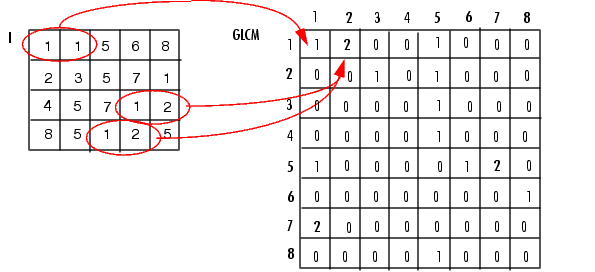GLCM表其实就是所有像素可能的组合，比如，GLCM（1,1）就是I中像素值为1和1的组合，GLCM（4,5）就是I中像素4和像素5的组合，GLCM（i,j）的值呢就是I中像素为i,像素为j的有有多少和相邻的成对点。这个相邻有个规则：就是f(x,y),f(x+a,y+b)相邻，就是只有x相隔a的单位，y相隔b个单位，我们认为是相邻的。
平时我们说相邻：B点在A点右边，其实就是这里的a=1,b=0,也就是f(x,y)和f(x+1,y+0)相邻。
于是就有了：
a=1,b=0 时我们就说水平相邻：也就是0度的时候
a=1,b=1 时我们就说对角相邻，也就是45度的时候
a=-1，b=1时 即135度
其他角度类似。
在a=1,b=0时：GLCM(1，1)=1；其实就是I中有几个1和1相邻（1个）（按上面的规则）GLCM(1，2)=2，几个1和2相邻（2个）。ok！
后面好多的性质，都是在把这个矩阵计算出来之后再在这个基础上运算的，那些就不难了！

附加理解2：
共生矩阵用两个位置的像素的联合概率密度来定义，它不仅反映亮度的分布特征，也反映具有同样亮度或者接近亮度的像素之间的位置分布特性，是有关图像亮度变化的二阶统计特征。它是定义一组纹理特征的基础。

由于纹理是由灰度在空间位置上反复出现而形成的，因而在图像空间中像个某距离的两像素之间会存在一定的灰度关系，即图像中灰度的空间相关特性。灰度共生矩阵就是一种通过研究灰度的空间相关特性来描述纹理的常用方法。

灰度直方图是对图像上单个像素具有某个灰度进行统计的结果，

 而灰度共生矩阵是对图像上保持某距离的两像素分别具有某灰度的状况进行统计得到的。

取图像(N×N)中任意一点 （x，y）及偏离它的另一点 （x+a，y+b），设该点对的灰度值为（g1，g2）。令点（x，y） 在整个画面上移动，则会得到各种 （g1，g2）值，设灰度值的级数为 k，则（g1，g2） 的组合共有 k^2;种。对于整个画面，统计出每一种（g1，g2）值出现的次数，然后排列成一个方阵，在用（g1，g2） 出现的总次数将它们归一化为出现的概率P（g1，g2），这样的方阵称为灰度共生矩阵。距离差分值（a，b） 取不同的数值组合，可以得到不同情况下的联合概率矩阵。（a，b）取值要根据纹理周期分布的特性来选择，对于较细的纹理，选取（1，0）、（1，1）、（2，0）等小的差分值。　　当 a=1，b=0时，像素对是水平的，即0度扫描；当a=0，b=1 时，像素对是垂直的，即90度扫描；当 a=1，b=1时，像素对是右对角线的，即45度扫描；当 a=-1，b=-1时，像素对是左对角线，即135度扫描。
这样，两个象素灰度级同时发生的概率，就将 （x，y）的空间坐标转化为“灰度对” （g1，g2）的描述，形成了灰度共生矩阵。（百度百科）
一幅图象的灰度共生矩阵能反映出图象灰度关于方向、相邻间隔、变化幅度的综合信息，它是分析图象的局部模式和它们排列规则的基础。

感觉差不多了吧！

2.灰度共生矩阵特征量（字写的不好，请见谅）

2.1对比度

度量 矩阵的值是如何分布和图像中局部变化的多少，反应了图像的清晰度和纹理的沟纹深浅。纹理的沟纹越深，反差越大，效果越清晰；反之，对比值小，则沟纹浅，效果模糊。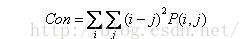2.2 能量

能量变换反映了图像灰度分布均匀程度和纹理粗细度。若灰度共生矩阵的元素值相近，则能量较小，表示纹理细致；若其中一些值大，而其它值小，则能量值较大。能量值大表明一种较均一和规则变化的纹理模式。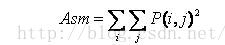2.3 熵

图像包含信息量的随机性度量。当共生矩阵中所有值均相等或者像素值表现出最大的随机性时，熵最大；因此熵值表明了图像灰度分布的复杂程度，熵值越大，图像越复杂。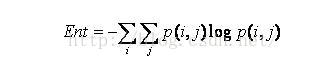2.4 逆方差

逆方差反映了图像纹理局部变化的大小，若图像纹理的不同区域间较均匀，变化缓慢，逆方差会较大，反之较小。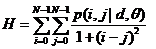2.5相关性

用来度量图像的灰度级在行或列方向上的相似程度，因此值得大小反应了局部灰度相关性，值越大，相关性也越大。3、代码

#!/usr/bin/python
# -*- coding: UTF-8 -*-
import cv2
import math

#定义最大灰度级数
gray_level = 16

def maxGrayLevel(img):
max_gray_level=0
(height,width)=img.shape
print ("图像的高宽分别为：height,width",height,width)
for y in range(height):
for x in range(width):
if img[y][x] > max_gray_level:
max_gray_level = img[y][x]
print("max_gray_level:",max_gray_level)
return max_gray_level+1

def getGlcm(input,d_x,d_y):
srcdata=input.copy()
ret=[[0.0 for i in range(gray_level)] for j in range(gray_level)]
(height,width) = input.shape

max_gray_level=maxGrayLevel(input)
#若灰度级数大于gray_level，则将图像的灰度级缩小至gray_level，减小灰度共生矩阵的大小
if max_gray_level > gray_level:
for j in range(height):
for i in range(width):
srcdata[j][i] = srcdata[j][i]*gray_level / max_gray_level

for j in range(height-d_y):
for i in range(width-d_x):
rows = srcdata[j][i]
cols = srcdata[j + d_y][i+d_x]
ret[rows][cols]+=1.0

for i in range(gray_level):
for j in range(gray_level):
ret[i][j]/=float(height*width)

return ret

def feature_computer(p):
#con:对比度反应了图像的清晰度和纹理的沟纹深浅。纹理越清晰反差越大对比度也就越大。
#eng:熵（Entropy, ENT）度量了图像包含信息量的随机性，表现了图像的复杂程度。当共生矩阵中所有值均相等或者像素值表现出最大的随机性时，熵最大。
#agm:角二阶矩（能量），图像灰度分布均匀程度和纹理粗细的度量。当图像纹理均一规则时，能量值较大；反之灰度共生矩阵的元素值相近，能量值较小。
#idm:反差分矩阵又称逆方差，反映了纹理的清晰程度和规则程度，纹理清晰、规律性较强、易于描述的，值较大。
Con=0.0
Eng=0.0
Asm=0.0
Idm=0.0
for i in range(gray_level):
for j in range(gray_level):
Con+=(i-j)*(i-j)*p[i][j]
Asm+=p[i][j]*p[i][j]
Idm+=p[i][j]/(1+(i-j)*(i-j))
if p[i][j]>0.0:
Eng+=p[i][j]*math.log(p[i][j])
return Asm,Con,-Eng,Idm

def test(image_name):
try:
img_shape=img.shape
except:
return

#这里如果用‘/’会报错TypeError: integer argument expected, got float
#其实主要的错误是因为 因为cv2.resize内的参数是要求为整数
img=cv2.resize(img,(img_shape//2,img_shape//2),interpolation=cv2.INTER_CUBIC)

img_gray=cv2.cvtColor(img, cv2.COLOR_BGR2GRAY)

glcm_0=getGlcm(img_gray, 1,0)
#glcm_1=getGlcm(src_gray, 0,1)
#glcm_2=getGlcm(src_gray, 1,1)
#glcm_3=getGlcm(src_gray, -1,1)
print(glcm_0)

asm,con,eng,idm=feature_computer(glcm_0)

return [asm,con,eng,idm]

if __name__=='__main__':
result = test("/home/images/2019-05-27/8/20190527_17_29_03_X1_Y2.jpg")
print(result)


展开全文• 图像处理库scikits-image已经支持计算灰度共生矩阵和提取GLCM的纹理属性contrast、dissimilarity、homogeneity、ASM、energy、correlation 首先了解一下灰度共生矩阵是什么，下面介绍摘自百度百科。...

图像处理库scikits-image已经支持计算灰度共生矩阵和提取GLCM的纹理属性contrast、dissimilarity、homogeneity、ASM、energy、correlation

首先了解一下灰度共生矩阵是什么，下面介绍摘自百度百科。https://baike.baidu.com/item/%E7%81%B0%E5%BA%A6%E5%85%B1%E7%94%9F%E7%9F%A9%E9%98%B5

灰度共生矩阵，指的是一种通过研究灰度的空间相关特性来描述纹理的常用方法。1973年Haralick等人提出了用灰度共生矩阵来描述纹理特征。
由于纹理是由灰度分布在空间位置上反复出现而形成的，因而在图像空间中相隔某距离的两像素之间会存在一定的灰度关系，即图像中灰度的空间相关特性。
取图像(N×N)中任意一点 （x，y）及偏离它的另一点 （x+a，y+b），设该点对的灰度值为 （g1，g2）。令点（x，y） 在整个画面上移动，则会得到各种 （g1，g2）值，设灰度值的级数为 k，则（g1，g2） 的组合共有 k 的平方种。对于整个画面，统计出每一种 （g1，g2）值出现的次数，然后排列成一个方阵，再用（g1，g2） 出现的总次数将它们归一化为出现的概率P（g1，g2） ，这样的方阵称为灰度共生矩阵。距离差分值（a，b） 取不同的数值组合，可以得到不同情况下的联合概率矩阵。（a，b） 取值要根据纹理周期分布的特性来选择，对于较细的纹理，选取（1，0）、（1，1）、（2，0）等小的差分值。
当 a=1，b=0时，像素对是水平的，即0度扫描；当a=0，b=1 时，像素对是垂直的，即90度扫描；当 a=1，b=1时，像素对是右对角线的，即45度扫描；当 a=-1，b=1时，像素对是左对角线，即135度扫描。
这样，两个象素灰度级同时发生的概率，就将 （x，y）的空间坐标转化为“灰度对” （g1，g2）的描述，形成了灰度共生矩阵。

灰度共生矩阵的特征
对比度（contrast）直接反映了某个像素值及其领域像素值的亮度的对比情况。如果偏离对角线的元素有较大值，即图像亮度值变化很快，则CON会有较大取值，这也符合对比度的定义。其中 。反映了图像的清晰度和纹理沟纹深浅的程度。纹理沟纹越深，其对比度越大，视觉效果越清晰；反之，对比度小，则沟纹浅，效果模糊。灰度差即对比度大的象素对越多，这个值越大。灰度公生矩阵中远离对角线的元素值越大，CON越大。

ASM 能量（angular second moment)也即每个矩阵元素的平方和。
如果灰度共生矩阵中的值集中在某一块（比如对连续灰度值图像，值集中在对角线；对结构化的图像，值集中在偏离对角线的位置），则ASM有较大值，若G中的值分布较均匀（如噪声严重的图像），则ASM有较小的值。
能量是灰度共生矩阵元素值的平方和，所以也称能量，反映了图像灰度分布均匀程度和纹理粗细度。如果共生矩阵的所有值均相等，则ASM值小；相反，如果其中一些值大而其它值小，则ASM值大。当共生矩阵中元素集中分布时，此时ASM值大。ASM值大表明一种较均一和规则变化的纹理模式。

自相关（correlation）其中自相关反应了图像纹理的一致性。如果图像中有水平方向纹理，则水平方向矩阵的COR大于其余矩阵的COR值。它度量空间灰度共生矩阵元素在行或列方向上的相似程度，因此，相关值大小反映了图像中局部灰度相关性。当矩阵元素值均匀相等时，相关值就大;相反，如果矩阵像元值相差很大则相关值小。

百度百科对应的只有上述三个特征的公式，下面的另外三个特征的公式来自http://tonysyu.github.io/scikit-image/api/skimage.feature.html

相异性（Dissimilarity）这里的P对应上面的G，levels对应k

计算对比度时，权重随矩阵元素与对角线的距离以指数方式增长，如果改为线性增长，则得到相异性。

同质性/逆差距（Homogeneity）测量图像的局部均匀性，非均匀图像的值较低，均匀图像的值较高。与对比度或相异性相反，同质性的权重随着元素值与对角线的距离而减小，其减小方式是指数形式的。

能量(Energy)是灰度共生矩阵各元素值的平方和，是对图像纹理的灰度变化稳定程度的度量，反应了图像灰度分布均匀程度和纹理粗细度。能量值大表明当前纹理是一种规则变化较为稳定的纹理。。

好了，下面开始开始实现上面的内容。通过skimage计算灰度共生矩阵并提取上述的六个纹理特征。

scikits-image库提供了两个模块，skimage.feature.greycomatrix(image, …[, …])计算灰度共生矩阵，skimage.feature.greycoprops(P[, prop])计算GLCM的纹理特征。

skimage.feature.greycomatrix(image, distances, angles, levels=256, symmetric=False, normed=False)

参数：
image：array_like of uint8

整数型输入图像。该图像将强制转换为uint8，因此最大值必须小于256。

distances：array_like

像素对距离偏移量列表。

angles：array_like

弧度的像素对角度列表。

级别：整数，可选

输入图像应在[0，levels-1]中包含整数，其中level表示所计数的灰度级数（对于8位图像，通常为256）。最大值为256。

对称：布尔型，可选

如果为True，则输出矩阵P [：，：，d，theta]是对称的。这是通过忽略值对的顺序来实现的，因此在给定偏移量遇到（i，j）时，将同时累积（i，j）和（j，i）。默认值为False。

标准化（归一化）：bool，可选

如果为True，则通过除以给定偏移量的累积共现总数，将每个矩阵P [：，：，d，theta]归一化。结果矩阵的元素总和为1。默认值为False。

举个例子演示一下
from skimage.feature import greycomatrix, greycoprops
import numpy as np
image = np.array([[0, 0, 1, 1],
[0, 0, 1, 1],
[0, 2, 2, 2],
[2, 2, 3, 3]], dtype=np.uint8)
#构建了一个44的数组
result = greycomatrix(image, , [0, np.pi/2], levels=4)#调用函数
#第一个参数是图像，第二个为距离1，第三个是扫描方向，这里加入了两个方向，0为向右扫描，np.pi/2,90°扫描即垂直扫描；最后一个levels=4即灰度级数。
运行这行代码，会返回一个四维的result结果。即灰度共生直方图，表示形式为p[i,j,d,theta],是灰度级j与灰度级i之间距离为d,角度为θ的情况下出现灰度级j的次数。生成的result是一个4x4x1x2的数组，因为原始输入的image是一个4
4的数组，距离d这里只计算了一个，角度计算了两个，所以是4x4x1x2

读取一下result数组
result[:, :, 0, 0] #距离d为1时，扫描角度为0时的result
array([[2, 2, 1, 0],
[0, 2, 0, 0],
[0, 0, 3, 1],
[0, 0, 0, 1]], dtype=uint32)
result[:, :, 0, 1] #距离d为1时，扫描角度为90°时的result
array([[3, 0, 2, 0],
[0, 2, 2, 0],
[0, 0, 1, 2],
[0, 0, 0, 0]], dtype=uint32)
这样一看难以理解，画了个图以便大家理解图中右边展示的是0°向右扫描，下边展示的是90°垂直扫描。生成的灰度共生矩阵里面的数是出现次数的统计。

尝试距离为2时的结果
result1 = greycomatrix(image,,[0, np.pi/2],levels=4)

result1[:, :, 0, 0]

array([[0, 4, 1, 0],
[0, 0, 0, 0],
[0, 0, 1, 2],
[0, 0, 0, 0]], dtype=uint32)

result1[:, :, 0, 1]

array([[1, 0, 3, 0],
[0, 0, 2, 2],
[0, 0, 0, 0],
[0, 0, 0, 0]], dtype=uint32)就画了下0°扫描的（0，1），垂直扫描的示意图就不画了，图已经有点乱了。

result2 = greycomatrix(image,[1,2,3,4],[0, np.pi/2,np.pi/4,np.pi*3/4],levels=4)# 距离为1，2，3，4,扫描方向为0°水平扫描，90°垂直扫描，45°右对角线扫描，135°左对角线扫描。#读取距离4，左对角线扫描的结果
result2[:, :, 3, 3]
array([[0, 0, 0, 0],
[0, 0, 1, 0],
[0, 0, 0, 0],
[0, 0, 0, 0]], dtype=uint32)

计算6个纹理特征

skimage.feature.greycoprops(P[, prop])

Parameters :
P : ndarray

Input array. P is the grey-level co-occurrence histogram for which to compute the specified property. The value P[i,j,d,theta] is the number of times that grey-level j occurs at a distance d and at an angle theta from grey-level i.

prop : {‘contrast’, ‘dissimilarity’, ‘homogeneity’, ‘energy’, ‘correlation’, ‘ASM’}, optional

The property of the GLCM to compute. The default is ‘contrast’.

Returns :
results : 2-D ndarray

2-dimensional array. results[d, a] is the property ‘prop’ for the d’th distance and the a’th angle.

参数：
P：ndarray

输入数组。P是用于计算指定属性的灰度共生直方图。值 P [i，j，d，θ]是灰度级j在距灰度级i的距离为d且角度为θ的角度出现的次数。

prop：{“对比度”，“相异性”，“同质性”，“能量”，“相关性”，“ ASM”}，可选

要计算的GLCM的属性。默认值为“对比度”。

返回值：
结果：2-D ndarray
二维数组。result [d，a]是第d个距离和第a个角度的属性’prop’。

image = np.array([[0, 0, 1, 1],
[0, 0, 1, 1],
[0, 2, 2, 2],
[2, 2, 3, 3]], dtype=np.uint8)
g = greycomatrix(image, [1, 2], [0, np.pi/2], levels=4,
normed=True, symmetric=True)#这里计算灰度共生矩阵，输出的矩阵P [：，：，d，theta]是对称的，并对结果进行了归一化
contrast = greycoprops(g, ‘contrast’)
contrast
array([[0.58333333, 1. ],
[1.25 , 2.75 ]])

dissimilarity = greycoprops(g, ‘dissimilarity’)
dissimilarity
array([[0.41666667, 0.66666667],
[1. , 1.5 ]])
#greycoprops()不能同时计算这六个特征，只能挨个计算。

官方文档见：http://tonysyu.github.io/scikit-image/api/skimage.feature.html

这两天因为任务刚接触这个，做了个整理，不足之处还请谅解。有错误之处还请指正，谢谢。

展开全文• 灰度共生矩阵的原理及代码实现（python） 1原理： 灰度共生矩阵（Gray Level Co-occurrence Matrix，GLCM）统计了灰度图中像素间的灰度值分布规律以区分不同的纹理。灰度共生矩阵中每个元素的值可以定义为(x, y)...机器学习
• 通过MATLAB运用灰度共生矩阵提取熵、能量、对比度、相关等特征
• 此文介绍了如何在一张图片中得到Glcm灰度共生矩阵,并基于Glcm的特征提取.带每一步的讲解 Glcm(Gray-level co-occurrence matrix) 灰度共生矩阵 原理：就是通过计算灰度图像得到它的共生矩阵，然后透过计算这个共生...计算机视觉
• 上面的三幅图像具有相同的颜色排列和像素强度，但具有不同的空间模式和分布，这些空间模式和分布无法通过平均值、中值或标准差等统计计算来识别，因此灰度共生矩阵 (GLCM) 为了解决这个问题而出现。 灰度共生矩阵...opencv
• 点击上方“小白学视觉”，选择加"星标"或“置顶”重磅干货，第一时间送达灰度共生矩阵灰度共生矩阵(Gray Level CO-Occurrence Matrix-GLCM)是图像特征分析与提...计算机视觉 opencv 人工智能 java
• ## 灰度共生矩阵（GLCM）附Python代码

万次阅读 多人点赞 2018-03-06 22:47:42
灰度共生矩阵（Gray-Level Co-occurrence Matrix，GLCM）统计了灰度图中像素间的灰度值分布规律以区分不同的纹理。 度共生矩阵可以定义为一个灰度为iii的像素点与另一个与之对应位置上的像素点的灰度值为jjj的概率...OpenCV
• 灰度共生矩阵特征分析法相邻某一间隔长度的两个像素，它们之间要么具有相同的灰度级，要么具有不同的灰度级，若能找出这样两个像素的联合分布的统计形式，对于图像的纹理分析很有意义。灰度共生矩阵(GLDM)的统计方法...
• 1 灰度共生矩阵原理 2 灰度共生矩阵特征量 2.1 对比度 2.2 能量 2.3 熵 2.4 逆方差 2.5 相关性 3 灰度共生矩阵特征量提取代码 1 灰度共生矩阵原理 灰度共生矩阵，指的是一种通过研究灰度的空间相关特性...
• 由于纹理是由灰度分布在空间位置上反复出现而形成的，因而在图像空间中相隔某距离的两象素之间会存在一定的灰度关系，即图像中灰度的空间相关特性。...灰度共生矩阵是像素距离和角度的矩阵函数，它通过计算图像中一定距
• 参考文献：Textural Features for Image Classification1...灰度共生矩阵（Gray-Level Co-occurrence Matrix，GLCM）统计了灰度图中像素间的灰度值分布规律以区分不同的纹理。 度共生矩阵可以定义为一个灰度为i ii的像
• 灰度共生矩阵所代表的含义是图像的灰度值...例如Haralick HaralickHaralick曾提出了14种基于灰度共生矩阵计算出来的统计量：能量、熵、对比度、均匀性、相关性、方差、和平均、和方差、和熵、差方差、差平均、差熵、...
• 灰度共生矩阵（Grey-Level Co-occurence Matrix，...对于纹理变化缓慢的图像，其灰度共生矩阵对角线上的数值较大；而对于纹理变化较快的图像，其灰度共生矩阵对角线上的数值较小，对角线两侧的值较大。 统计量：Ha...
• 展开全部%**************************************************************************% 图像检索——纹理特征%基于共生矩阵32313133353236313431303231363533e58685e5aeb931333264636265纹理特征提取，d=1,θ=0°...java 纹理
• ## 灰度共生矩阵(GLCM)

千次阅读 2020-04-13 17:08:34
灰度共生矩阵(GLCM) 算法简介 灰度共生矩阵法(GLCM, Gray - level co - occurrence...灰度共生矩阵能反映图像灰度关于方向、相邻间隔、变化幅度等综合信息，它是分析图像的局部模式和它们排列规则的基础。 （灰度共...机器学习 算法
• 基于灰度共生矩阵的图形纹理检测及路面状况的SVM分类实现 项目源码： 文章目录基于灰度共生矩阵的图形纹理检测及路面状况的SVM分类实现灰度共生矩阵的特征参数实现过程分类结果设计总结 图像的特征提取是图像的识别...SVM MATLAB
• Reference 利用python的skimage计算灰度共生矩阵 灰度共生矩阵（GLCM）附Python代码 灰度共生矩阵（附python代码） 灰度共生矩阵原理 灰度共生矩阵的生成和理解 灰度共生矩阵的理解 图像特征提取——灰度共生矩阵...
• 文章目录0，感谢1，灰度共生矩阵(Gray Level Cooccurrence Matrix)1.1初识1.2Opencv实现 0，感谢 学习记录，不当之处多多指教，在此感谢以下博主 灰度共生矩阵的原理及实现(特征提取)-OpenCV 灰度共生矩阵的生成...
• 灰度共生矩阵是像素距离和角度的矩阵函数，它通过计算图像中一定距离和一定方向的两点灰度之间的相关性，来反映图像在方向、间隔、变化幅度及快慢上的综合信息。 使用方法： glcm = graycomatrix(I) glcms = ...image matrix
• 版权声明：本文为博主原创文章，未经博主允许不得转载。 ... MATLAB中灰度共生矩阵graycomatrix()函数用法 glcm = graycoma...
• 概念 编辑 由于纹理是由灰度分布在空间位置上反复出现而形成的，因而...灰度直方图是对图像上单个象素具有某个灰度进行统计的结果，而灰度共生矩阵是对图像上保持某距离的两象素分别具有某灰度的状况进行统计得到的。
• 灰度共生矩阵 1matlab实现，来自网络转载 %************************************************************************** % 图像检索——纹理特征 %基于共生矩阵纹理特征提取，d=1,θ=0°,45°,90°,135°共四个...
• 鉴于最近复习线性代数计算量较大，且1800答案常常忽略一些逆阵、行列式的计算答案，故用python写出矩阵的简单计算程序，便于检查出错的步骤。 1、行列式 可自行更改阶数from numpy import * # 求行列式 ,建议：取...
• 图像点处理1.1 图像翻转1.2 幂运算和对数运算2. 直方图处理 说明 本文记录数字图像处理相关问题。导入的依赖如下： import cv2 import matplotlib import matplotlib.pyplot as plt import numpy as np from PIL ...
• 又叫做灰度共现矩阵 ...计算方式 ...对于精度要求高且纹理细密...我们来看，matlab内置工具箱中的灰度共生矩阵的生成函数graycomatrix（gray-level co-occurrence matrix）对方向的说明： 如上图所示，方...
• #这一步类似于数据压缩，因为8位图像含有256个灰度级，这样会导致计算灰度共生矩阵是的计算量过大，因此将其进行压缩成16级，将灰度进行分区 bins = np.array([0, 16, 32, 48, 64, 80, 96, 112, 128, 144, 160, ...python